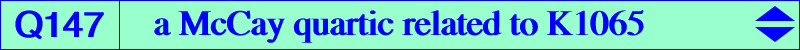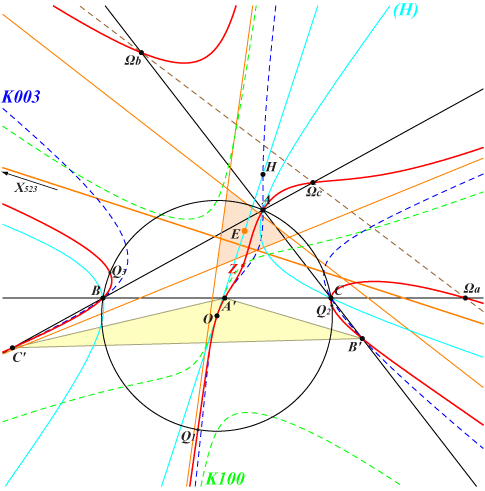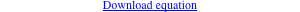too complicated to be written here. Click on the link to download a text file.X(3), X(523), X(5000), X(5001), X21444) vertices of the cevian triangle A'B'C' of X(3) centers Ωa, Ωb, Ωc of the Apollonius circles other points belowQ147 is a quartic related to the mapping 𝚹 : P → aQ described in page K1065. It is indeed the locus of M such that the circumcenter X(3) of ABC, P and 𝚹(P) are collinear. It follows that Q146 must contain the 6 singular points and the 7 fixed points of 𝚹, among them Z always real. Q147 has four real asymptotes : one is perpendicular to the Euler line and three are parallel to those of the McCay cubic K003. These bound an equilateral triangle with center E on the Euler line, also on the lines {399,14933}, {1624,5655}, {5467,22115}. Q147 is tangent to the Euler line at X(3) and meets this latter line again at two other points (imaginary when ABC is not acute) X(5000), X(5001) that also lie on Q146 and on the circum-hyperbola (H) passing through X(6), X(232), X(250), X(262), X(264), X(325), X(511), X(523), X(842), X(1485), X(2065), etc.